Net Force Ranking Tasks - Questions

The Net Force Ranking TasksConcept Builder is comprised of 36 questions. The questions are divided into 12 different Question Groups. Questions in the same group are rather similar to one another. The Concept Builder is coded to select at random a question from each group until a student is successful with that group of questions.

There are three levels of difficulty by which the student can progress through the Concept Builder. Those three difficulty levels are differentiated as follows:

• Apprentice Level: Question Groups 1-4 ... Students rank four situations.
• Masters Level: Question Groups 5-8 ... Students rank six situations.
• Wizard Level: Question Groups 9-12 ... Students rank eight situations.

The questions from each group are shown below. Teachers are encouraged to view the questions in order to judge which level of difficulty is most appropriate for their classes. We recommend providing students two or more options. For instance, it would be profitable for a student to complete the Concept Builder at the Apprentice Level in order to gain some confidence and then to be challenged to complete the activity at the Masters Level.

The Physics Classroom grants teachers and other users the right to print these questions for private use. Users are also granted the right to copy the text and modify it for their own use. However, this document should not be uploaded to other servers for distribution to and/or display by others. The Physics Classroom website should remain the only website or server from which the document is distributed or displayed. We also provide a PDF that teachers can use under the same conditions. We have included a link to the PDF near the bottom of this page.

Net Force (and Acceleration) Ranking Tasks

Question Group 1
Question 1
Rank the objects according to net force; use 1 for the most negative and 4 for the most positive.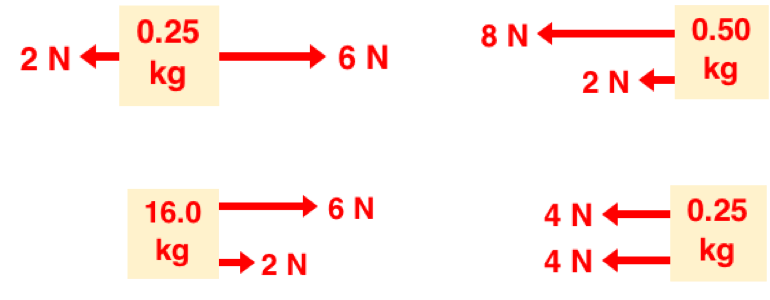Question 2
Rank the objects according to net force; use 1 for the most negative and 4 for the most positive.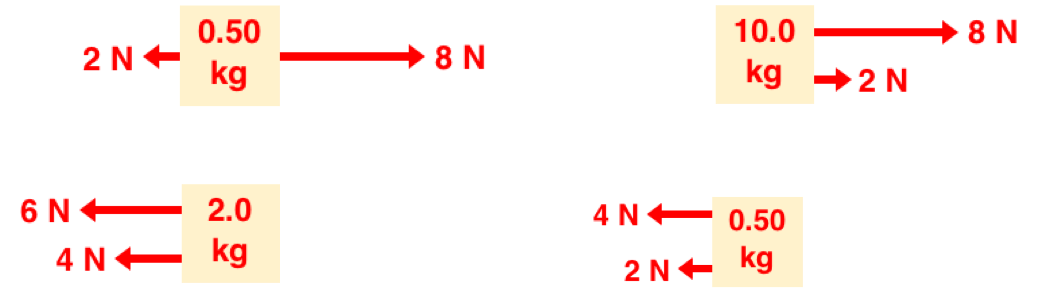Question 3
Rank the objects according to net force; use 1 for the most negative and 4 for the most positive.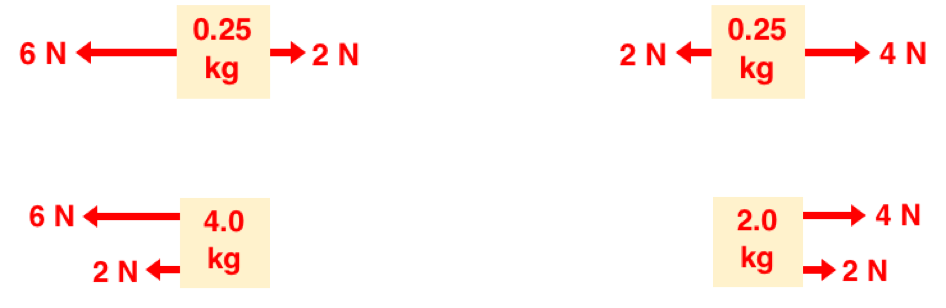Question Group 2
Question 4
Rank the objects according to net force; use 1 for the most negative and 4 for the most positive.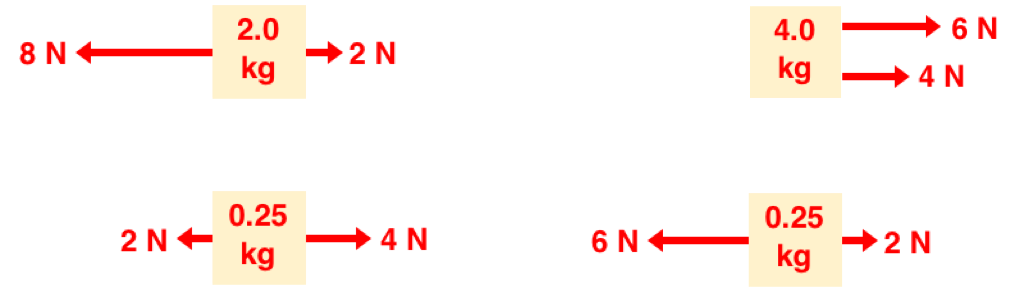Question 5
Rank the objects according to net force; use 1 for the most negative and 4 for the most positive.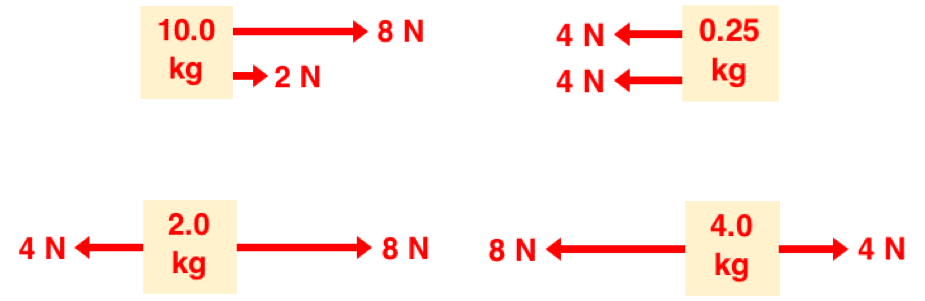Question 6
Rank the objects according to net force; use 1 for the most negative and 4 for the most positive.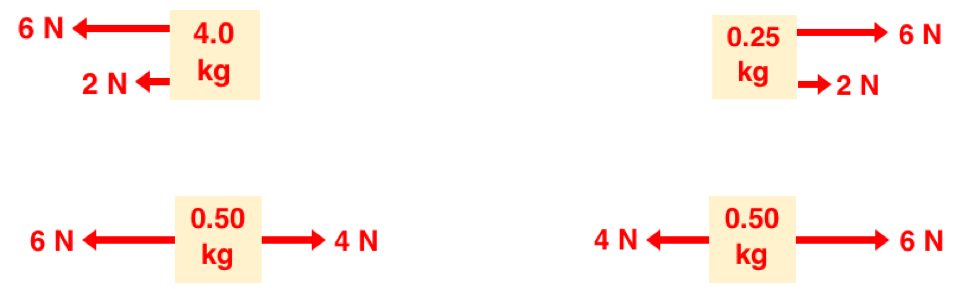Question Group 3
Question 7
Rank the objects according to acceleration; use 1 for the most negative and 4 for the most positive.Question 8
Rank the objects according to acceleration; use 1 for the most negative and 4 for the most positive.Question 9
Rank the objects according to acceleration; use 1 for the most negative and 4 for the most positive.Question Group 4
Question 10
Rank the objects according to acceleration; use 1 for the most negative and 4 for the most positive.Question 11
Rank the objects according to acceleration; use 1 for the most negative and 4 for the most positive.Question 12
Rank the objects according to acceleration; use 1 for the most negative and 4 for the most positive.Master Difficulty Level
Question Group 5
Question 13
Rank the objects according to net force; use 1 for the most negative and 6 for the most positive.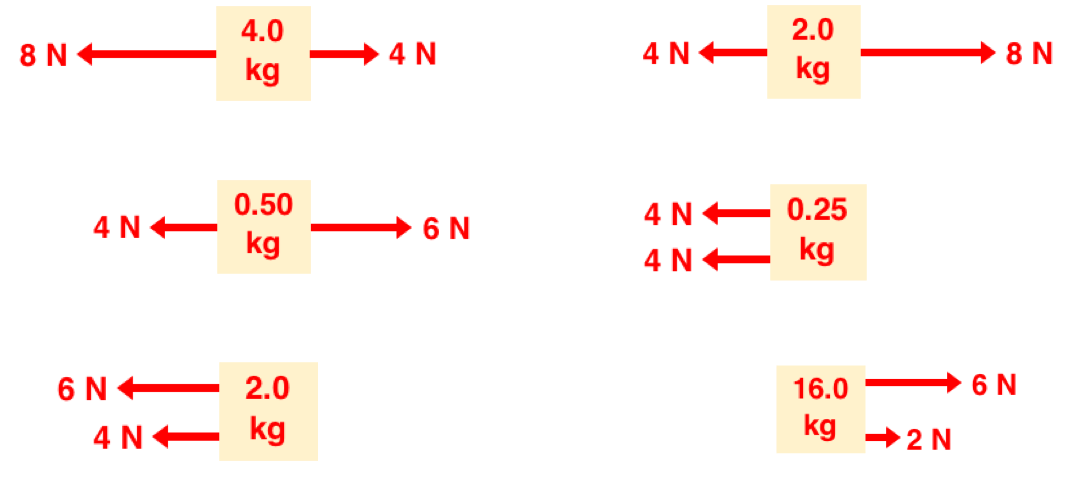Question 14
Rank the objects according to net force; use 1 for the most negative and 6 for the most positive.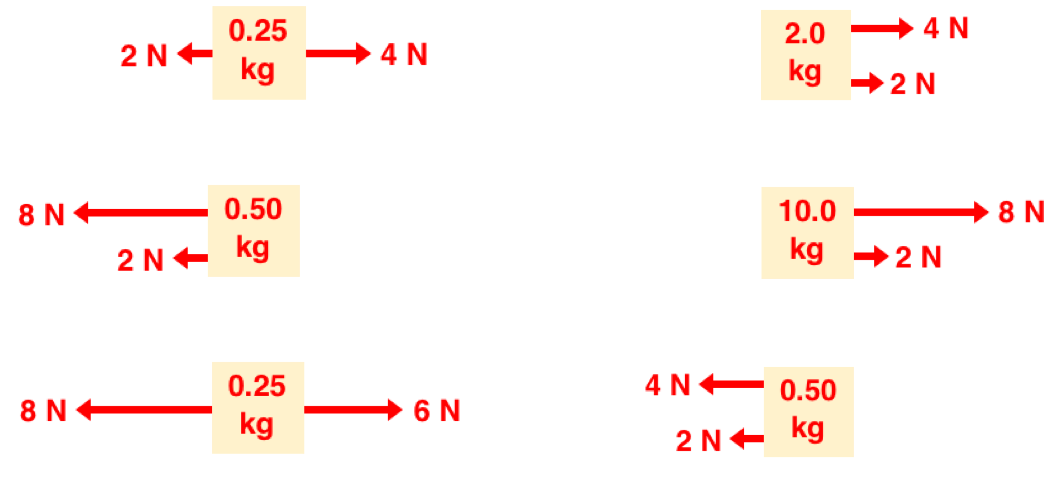Question 15
Rank the objects according to net force; use 1 for the most negative and 6 for the most positive.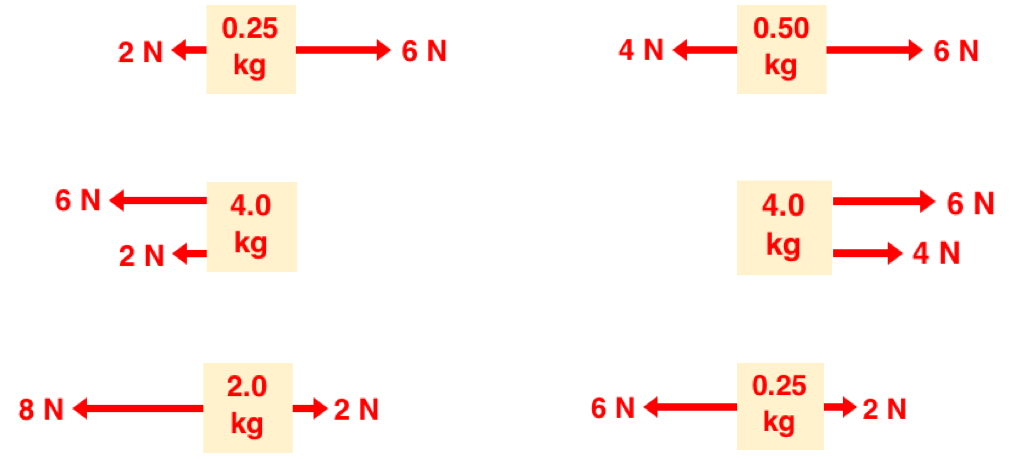Question Group 6
Question 16
Rank the objects according to net force; use 1 for the most negative and 6 for the most positive.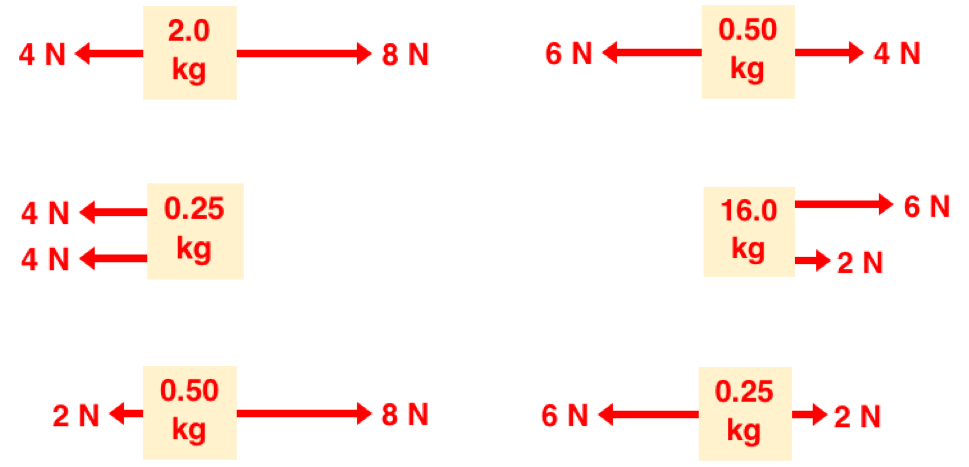Question 17
Rank the objects according to net force; use 1 for the most negative and 6 for the most positive.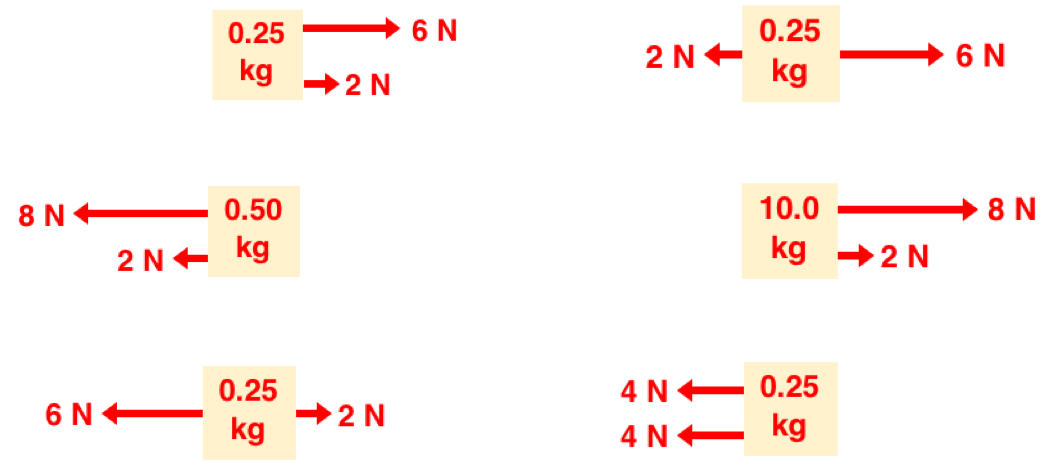Question 18
Rank the objects according to net force; use 1 for the most negative and 6 for the most positive.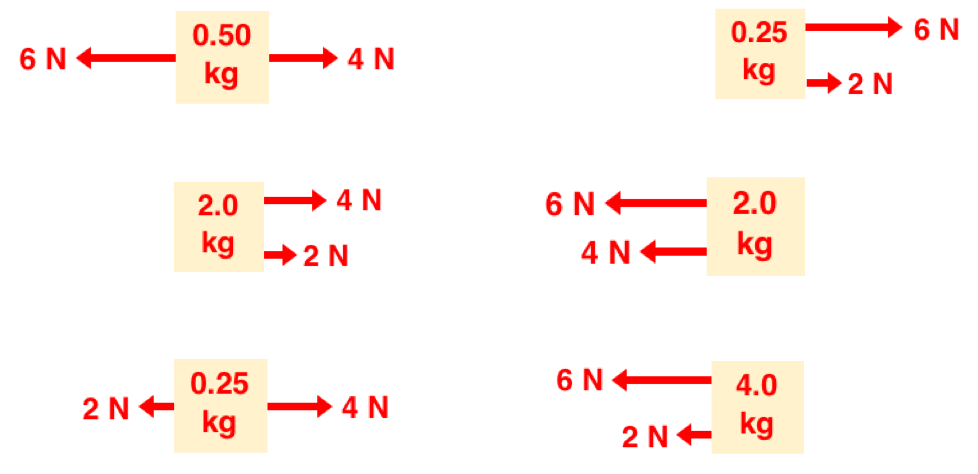Question Group 7
Question 19
Rank the objects according to acceleration; use 1 for the most negative and 6 for the most positive.Question 20
Rank the objects according to acceleration; use 1 for the most negative and 6 for the most positive.Question 21
Rank the objects according to acceleration; use 1 for the most negative and 6 for the most positive.Question Group 8
Question 22
Rank the objects according to acceleration; use 1 for the most negative and 6 for the most positive.Question 23
Rank the objects according to acceleration; use 1 for the most negative and 6 for the most positive.Question 24
Rank the objects according to acceleration; use 1 for the most negative and 6 for the most positive.Wizard Difficulty Level
Question Group 9
Question 25
Rank the objects according to net force; use 1 for the most negative and 8 for the most positive.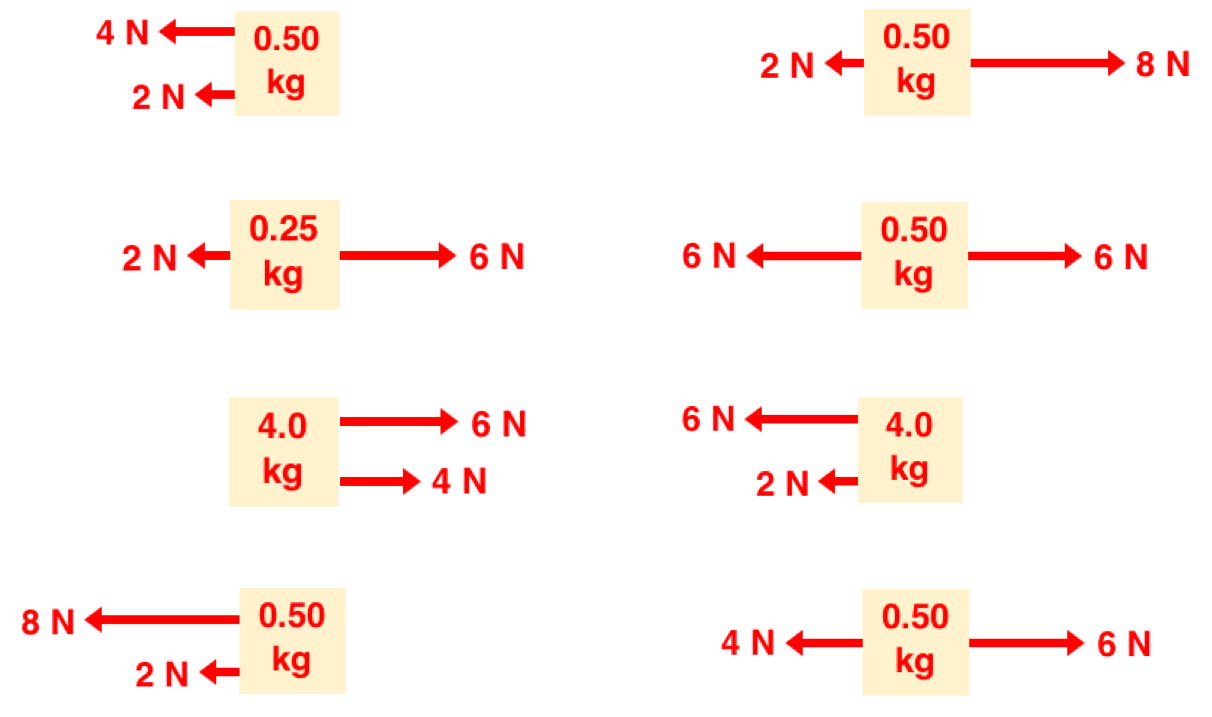Question 26
Rank the objects according to net force; use 1 for the most negative and 8 for the most positive.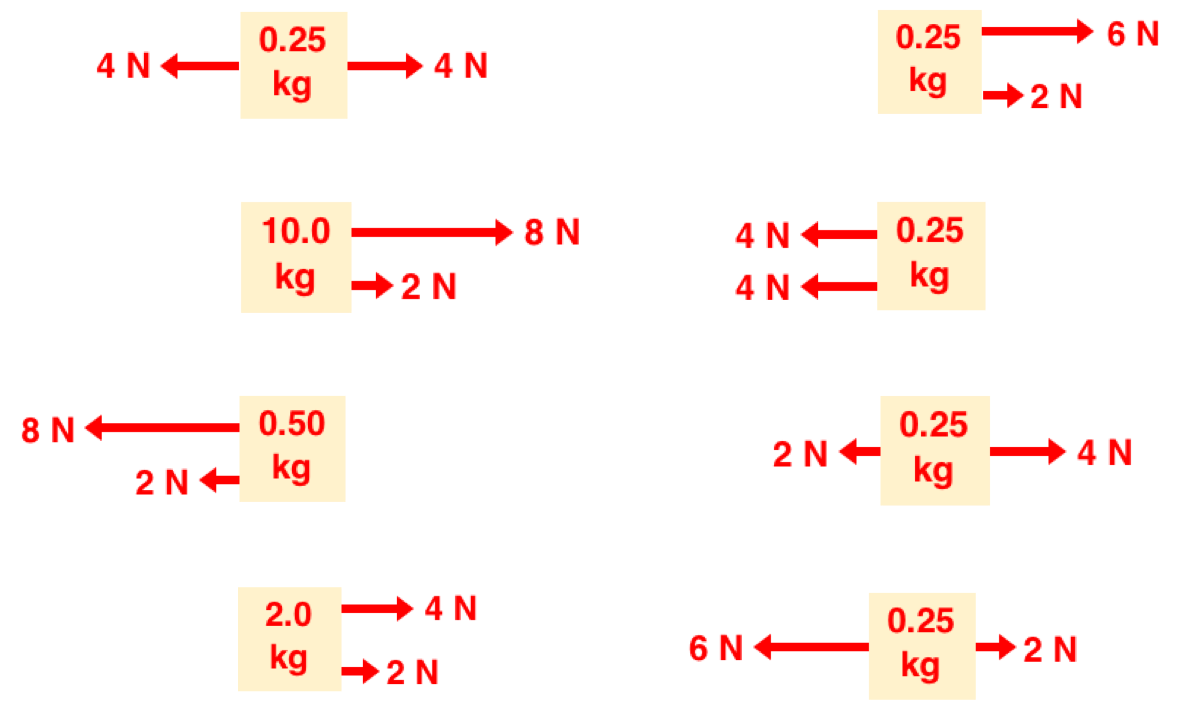Question 27
Rank the objects according to net force; use 1 for the most negative and 8 for the most positive.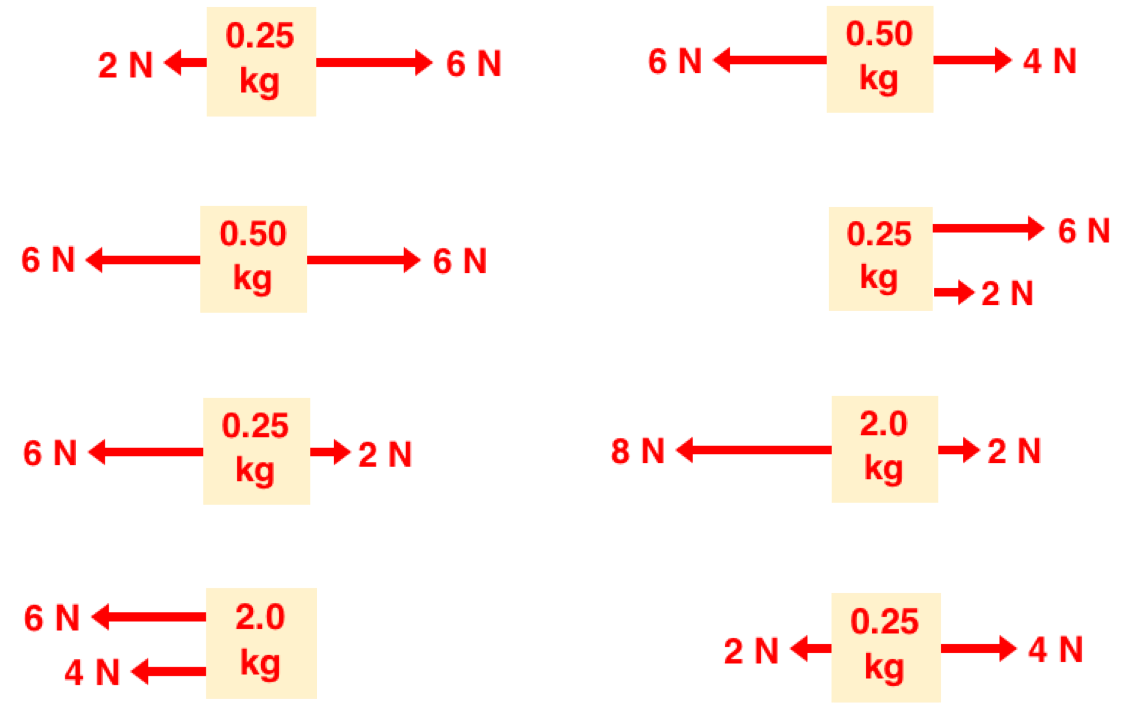Question Group 10
Question 28
Rank the objects according to net force; use 1 for the most negative and 8 for the most positive.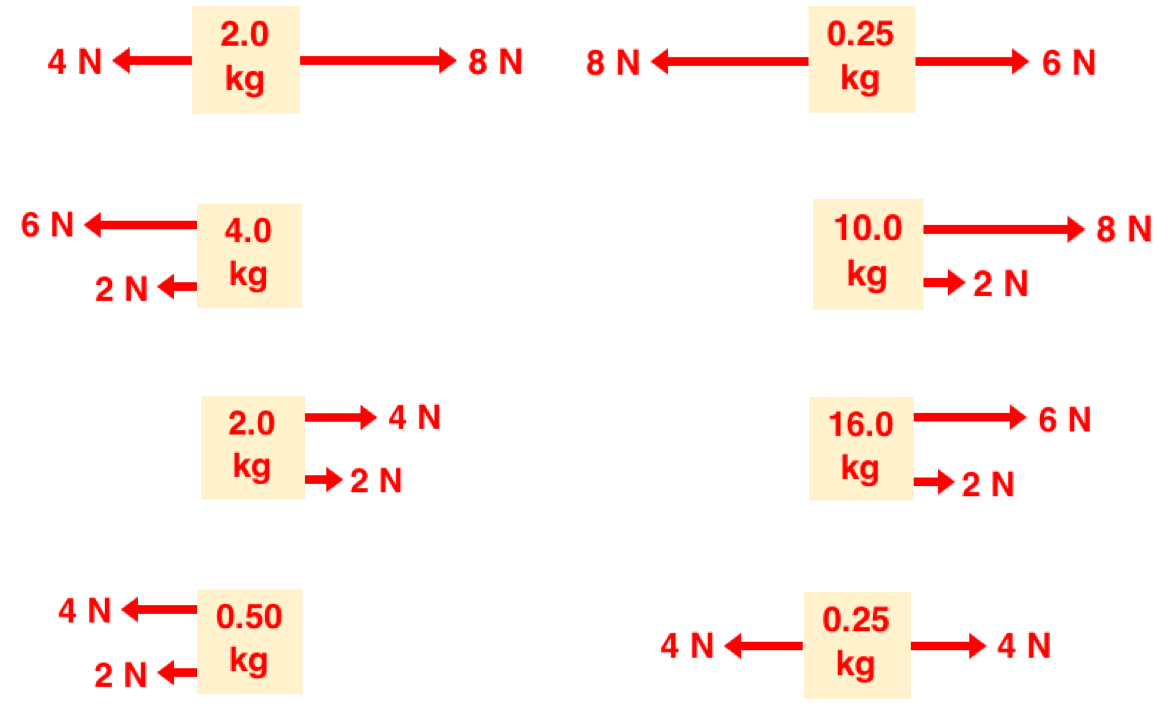Question 29
Rank the objects according to net force; use 1 for the most negative and 8 for the most positive.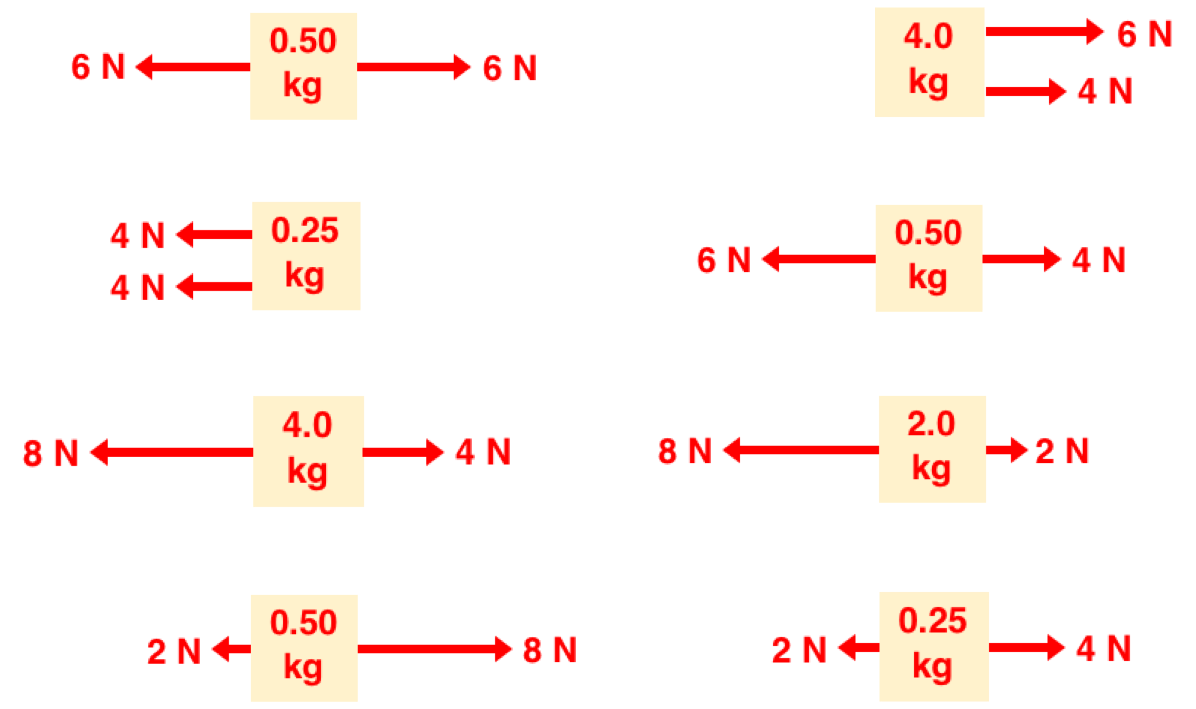Question 30
Rank the objects according to net force; use 1 for the most negative and 8 for the most positive.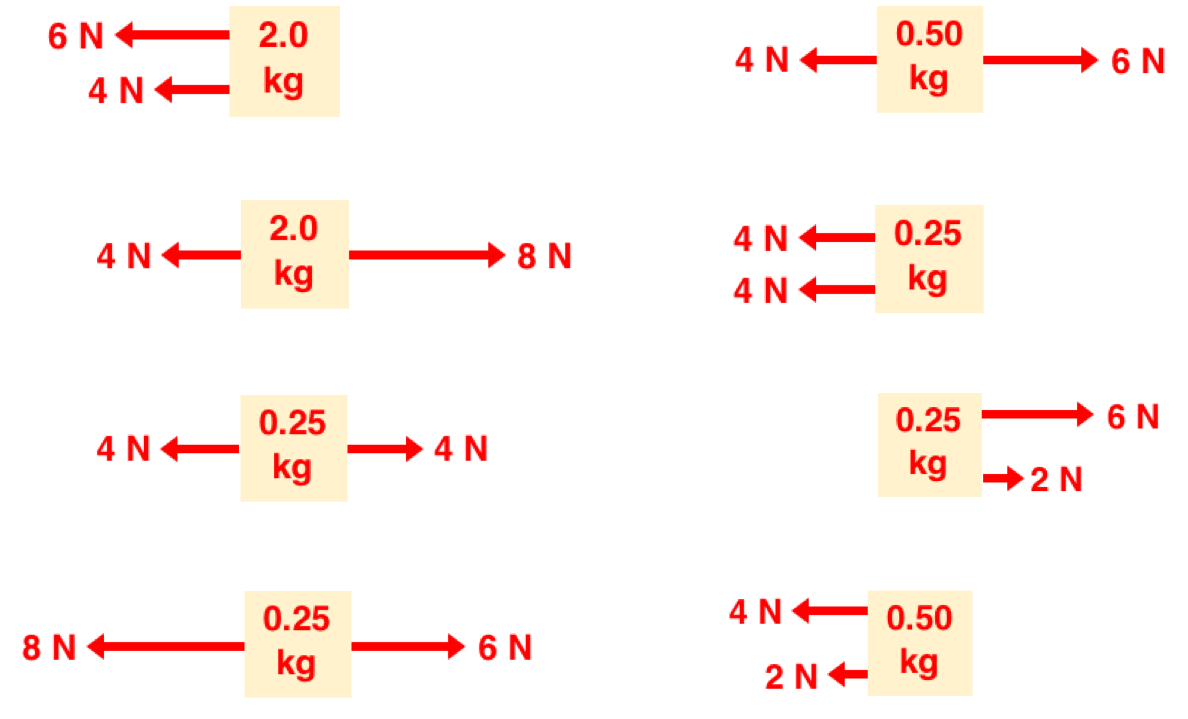Question Group 11
Question 31
Rank the objects according to acceleration; use 1 for the most negative and 8 for the most positive.Question 32
Rank the objects according to acceleration; use 1 for the most negative and 8 for the most positive.Question 33
Rank the objects according to acceleration; use 1 for the most negative and 8 for the most positive.Question Group 12
Question 34
Rank the objects according to acceleration; use 1 for the most negative and 8 for the most positive.Question 35
Rank the objects according to acceleration; use 1 for the most negative and 8 for the most positive.Question 36
Rank the objects according to acceleration; use 1 for the most negative and 8 for the most positive.A special thanks to Professor Brian Frank (@brianwfrank) for inspiring this idea.

View:  Questions in PDF Format

Visit: Concept Builder​Teacher Notes